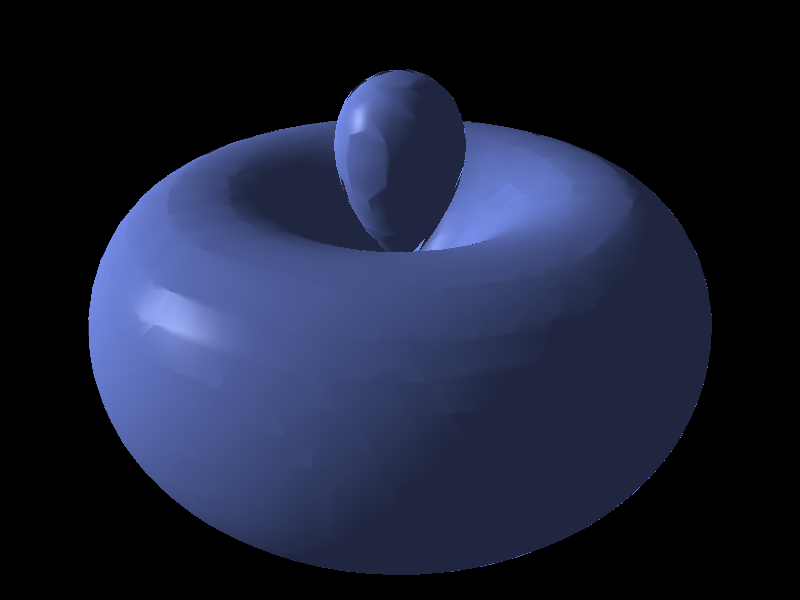# Isosurface Visual#

This example demonstrates the use of the Isosurface visual.```Generating scalar field..
```

```import sys
import numpy as np

from vispy import app, scene

# Create a canvas with a 3D viewport
canvas = scene.SceneCanvas(keys='interactive')

# Define a scalar field from which we will generate an isosurface
def psi(i, j, k, offset=(25, 25, 50)):
x = i-offset
y = j-offset
z = k-offset
th = np.arctan2(z, (x**2+y**2)**0.5)
r = (x**2 + y**2 + z**2)**0.5
a0 = 1
ps = ((1./81.) * 1./(6.*np.pi)**0.5 * (1./a0)**(3/2) * (r/a0)**2 *
np.exp(-r/(3*a0)) * (3 * np.cos(th)**2 - 1))
return ps

print("Generating scalar field..")
data = np.abs(np.fromfunction(psi, (50, 50, 100)))

# Create isosurface visual
surface = scene.visuals.Isosurface(data, level=data.max()/4.,
parent=view.scene)
surface.transform = scene.transforms.STTransform(translate=(-25, -25, -50))

# Add a 3D axis to keep us oriented
axis = scene.visuals.XYZAxis(parent=view.scene)

# Use a 3D camera
# Manual bounds; Mesh visual does not provide bounds yet
# Note how you can set bounds before assigning the camera to the viewbox
cam = scene.TurntableCamera(elevation=30, azimuth=30)
cam.set_range((-10, 10), (-10, 10), (-10, 10))
view.camera = cam

if __name__ == '__main__':
canvas.show()
if sys.flags.interactive == 0:
app.run()
```

Total running time of the script: ( 0 minutes 1.616 seconds)

Gallery generated by Sphinx-Gallery